# Surds and Indices - Quantitative Aptitude Notes | Study Quantitative Aptitude (Quant) - CAT

## CAT: Surds and Indices - Quantitative Aptitude Notes | Study Quantitative Aptitude (Quant) - CAT

The document Surds and Indices - Quantitative Aptitude Notes | Study Quantitative Aptitude (Quant) - CAT is a part of the CAT Course Quantitative Aptitude (Quant).
All you need of CAT at this link: CAT

Q 1. What will come in place o f question mark in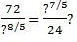a. 12
b. 6
c. 16
d. 8

Explanation:.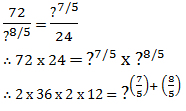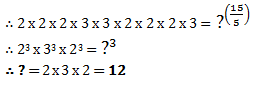Q 2. What is value of (0.000001) ?

a. 0.000001
b. 0.001
c. 1/100
d. 0.1

Explanation: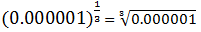If a cube has 6 decimals, then its cube
root will have 6/3 = 2 decimals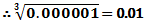Q 3. What will be value of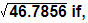(684)2= 467856?

a. 6.84
b. 0.0684
c. 0.684
d. 0.000684

Explanation:

I f a square has 4 decimals, then its
square root will have 4/2 = 2 decimals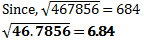Q 4. If value of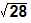is approximately 5.2915, then value of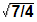is approximately

a. 1.2000
b. 0.5687
c. 1.3228
d. 1.4652

Explanation: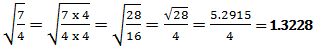Q 5. How many zeroes are there in 200010?

a. 10
b. 30
c. 20
d. 15

Explanation:

200010 = 2000 x ... 10 times
2000 = 2 x 1000 i.e it has 3 zeroes.

So that means 3 zeroes x 10 times= 30 zeroes

Q 6. What is value of (36)?

a. 2
b.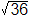c. 1
d.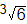ANSWER:Explanation: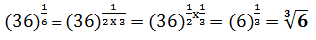Q 7. What is value of M in (p/q)2M+2 = (q/p)9-M

a. 6
b. 5
c. -7/2
d. -11

Explanation: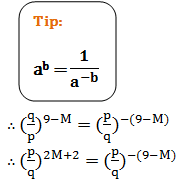Since the base is same i.e. p/q, we can equate the powers of both
∴ 2M+2 = -(9-M)

∴ 2M+2 = -9+M

∴ M = -11

Q 8. What is value of 22(-2)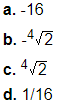ANSWER: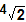Explanation: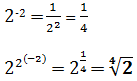Q 9. What is value of 2(-2)2

a. 1/16
b. 1/8
c. 32
d. 16

Explanation: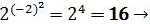Negative number raised to even power gives positive answer

Q 10. There are four expressions given below separated by ‘=’ sign. Which one of the 4 expressions is not the same as the other three?
62 x 6 = (61)3 = 23+33 = 83 - 142 - 102

a. (61)3
b. 83 - 142 - 102
c. 23+33
d. All are same

Explanation:

This is very easy to solve
The first expression is 62 x 6 = 63 = 216.

The second expression is (61)3 = 61 x 3 = 63 = 216
The third expression is 23+33 = 8+27 = 35 = ≠ other two

Question 11: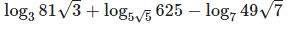= ?

a) 5
b) 6
c) 7
d) None of these
Explanation: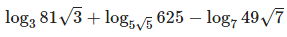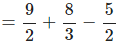= 14/3

Question 12: Find the value of x in 2|5x - 6| = 83x - 2
a) 2/3
b) 6/7
c) 9/5
d) cannot be determined
Explanation: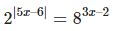Taking log on both sides we get,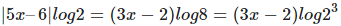|5x – 6| = 3(3x – 2) = 9x – 6
When x > 6/5, |5x – 6| = 5x – 6
Thus, 5x – 6 = 9x – 6
5x = 9x which is not possible
When x < 6/5, |5x – 6| = 6 – 5x
Thus, 6 – 5x = 9x – 6
14x = 12
x = 6/7

Question 13: If log152 729 = x, then what is the value of log81 5625 ?

a)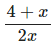b)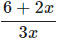c)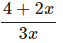d)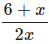Explanation:  log125 729
= log5 9
= x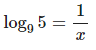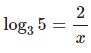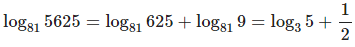=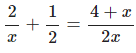Question 14: Find the number of digits in the number 240127 when written in base 7.
a) 117
b) 181
c) 98
d) 109

Explanation:
The formula for finding the number of digits in a number ‘m’ in base ‘n’ is:
[logn(m)] + 1 , where [A] is greatest integer less than or equal to A.
2401 = 74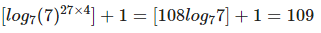Question 15: What is the value of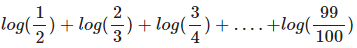a) -2
b) -1
c) 0
d) None of these

Explanation: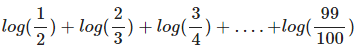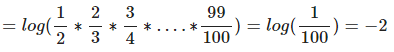Question 16: If  32x+3-244*3x = -9, then which of the following statements is true?
a) ‘x’ is a positive number
b) ‘x’ is a negative number
c) ‘x’ can be either a positive number or a negative number
d) None of the above

Explanation:
The equation can be written as follow: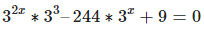Let 3x = t
⇒ 27t2 - 244t + 9 = 0
⇒ 27t2 - 243t-t + 9 = 0
⇒ 27t(t - 9)-1(t - 9) = 0
t = 9 or t = 1/27
⇒ 3x = 32 or 3x = 3-3
So, x = 2 or x = -3
So, ‘x’ can be either a positive number or a negative number. Option c) is the correct answer

Question 17: If x,y are natural numbers, log8+log5 = log(x+y)+log(x-y) and log8+log11 = log(x-y)+log(1+xy), then find the value of (x3 - y3)
a) 325
b) 316
c) 643
d) 602

Explanation:
log(x+y)(x-y) = log40
(x+y)(x-y) = 40
x+y = 10 and x-y = 4 or x+y = 20 and x-y = 2
x = 7 and y = 3 or x = 11 and y = 9.
log88 = log(x-y)(1+xy)
x = 7 and y = 3 satisfy the equation.

(x3 - y3) = 73 - 33 = 316

Question 18: If log16 5 = x and log3 = y, find the value of log3 6 in terms of x and y?
a)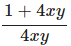b)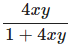c)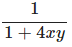d) 1 + 4xy
Explanation:

log16 5 = x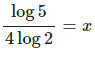log5 3 = y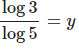We have to find the value of log3 6

= 1+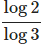= 1+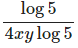= 1+1/4xy =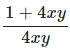Question 19: Find the value of log1010 + log10102 + ..... +log1010n

a)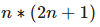b)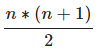c)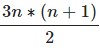d)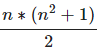Explanation: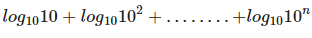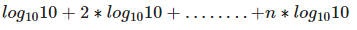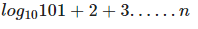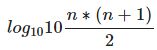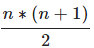Question 20: If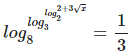. Find x
a) 512
b) 289
c) 170
d) None of these
Explanation: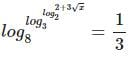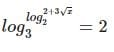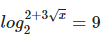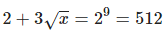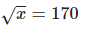⇒ x = 28900
Since there is no such option, the correct option to choose is D – None of these.

Logarithms, Surds and Indices questions will be asked in CAT quantitative aptitude section,

The document Surds and Indices - Quantitative Aptitude Notes | Study Quantitative Aptitude (Quant) - CAT is a part of the CAT Course Quantitative Aptitude (Quant).
All you need of CAT at this link: CATUse Code STAYHOME200 and get INR 200 additional OFF

## Quantitative Aptitude (Quant)

153 videos|129 docs|131 tests

### How to Prepare for CAT

Read our guide to prepare for CAT which is created by Toppers & the best Teachers

Track your progress, build streaks, highlight & save important lessons and more!

,

,

,

,

,

,

,

,

,

,

,

,

,

,

,

,

,

,

,

,

,

;